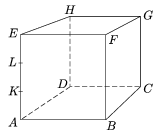g New exercises and problems in Mathematics - January 2004Mathematical and Physical Journal
for High Schools
Issued by the MATFUND Foundation
 Already signed up? New to KöMaL?

# New exercises and problems in MathematicsJanuary 2004## New exercises

Maximum score for each exercise (sign "C") is 5 points.

C. 745. Are there 2004 positive integers whose sum is equal to their product?

C. 746. How many numbers of the form $\displaystyle \overline{ababab}$ are divisible by a) 217; b) 218?

C. 747. The base of an isosceles triangle is unity and the lengths of its legs is b. How long is the base of the isosceles triangle whose legs enclose an angle equal to the angles lying on the base of the first triangle and whose legs are one unit long?

C. 748. Solve the following equation on the set of integers: $\displaystyle \sin\left(\frac{\pi}{3}\, \big(x-\sqrt{x^2-3x-12}\,\big)\right)=0$.

C. 749. The edges of the cube in the Figure (see page 39) are 6 units long. The points K and L trisect the edge AE. The cube is cut into pieces by the planes LHG and KFG. Find the volume of the part containing vertex B.## New problems

The maximum scores for problems (sign "B") depend on the difficulty. It is allowed to send solutions for any number of problems, but your score will be computed from the 6 largest score in each month.

B. 3692. We have two boxes of pebbles. One box contains p pebbles and the other one contains q. We are allowed to take either one pebble out of each box or multiply the number of pebbles in one box by three. Is it possible to empty both boxes by repeating these two moves if ap=100, q=200; bp=101, q=200? (4 points)

B. 3693. When a positive integer k divided by the prime number p, the remainder is 6. If the number 1000-k is divided by p, the remainder is the same, and, furthermore, 10 000-k is divisible by p. What is the prime number p? (3 points) (Suggested by G. Bakonyi, Budapest)

B. 3694. Given the convex quadrilateral ABCD, construct the four lines passing through the vertex A that divide the area of the quadrilateral into five equal parts. (3 points)

B. 3695. Let n be a positive integer. Prove that 5n-8n2+4n-1 is divisible by 64. (3 points)

B. 3696. The endpoints of a number of open rays lying on a line divide the line into parts. Prove that there are at most q+1 parts that are covered by exactly q rays. (4 points)

B. 3697. Solve the following equation:

$\displaystyle x^2+\left(\frac{5x}{x-5}\right)^{\!\!2}=11.$

(4 points)

B. 3698. Given the third vertices of the equilateral triangles drawn over the sides of a quadrilateral outside'' construct the triangle. No discussion of the solutions is expected this time. (5 points)

B. 3699. The point P lies on the diameter AB of a unit circle. A chord through P intersects the circle at the points C and D. What is the maximum area of the quadrilateral ABCD? (5 points)

B. 3700. Prove that if x1,x2,...,xn are positive numbers then

$\displaystyle \frac{x_1^3}{x_1^2+x_1x_2+x_2^2}+ \frac{x_2^3}{x_2^2+x_2x_3+x_3^2}+\ldots+ \frac{x_n^3}{x_n^2+x_nx_1+x_1^2}\ge\frac{1}{3}(x_1+x_2+\ldots+ x_n).$

(5 points) (Gillis-Turán Competition)

B. 3701. Let P denote an arbitrary point of a cube. Prove that there are at least six tetrahedra that contain P and the vertices of which are vertices of the cube. (4 points)Maximum score for each advanced problem (sign "A") is 5 points.

A. 335. By only using identical integers, the four basic operations and brackets, Steve is preparing further positive integers. For example:

$\displaystyle \frac{100\cdot100}{\frac{100}{100}- \frac{100}{100+100}}=20\;000$

Playing around, he maneged to produce a positive integer less than $\displaystyle \frac{|n|}{2^k}$ using the number n exactly k times. Prove that the expression found by Steve was an identity, that is, he would have obtained the same result by plugging any other number for n in the expression (unless the expression is undefined). (Based on the idea of L. Szobonya)

A. 336. Let a denote an odd number and let b denote an even number, such that a2+b2=p is a prime number. Prove that the equation x2-py2=a has an integer solution.

A. 337. The lines bounding a number of open half planes in the plane divide the plane into convex domains. Find a quadratic expression C(q) such that it is true for any integer q$\displaystyle \ge$1 that if the half planes cover each point of the plane at least q times then the set of points covered exactly q times is the union of at most C(q) domains. (Schweitzer Competition, 2003)